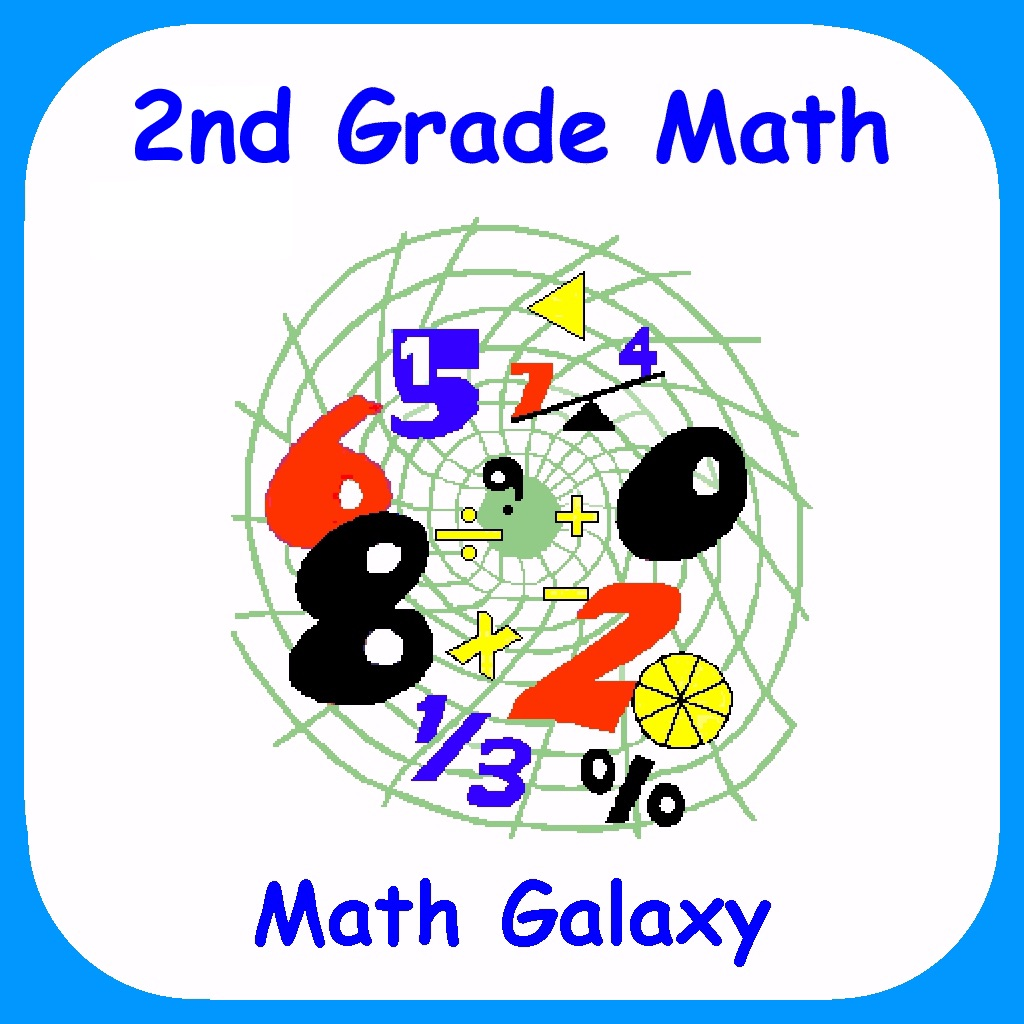2nd Grade Math - Math Galaxy# 2nd Grade Math - Math Galaxy

3.1 for iPhone, iPad
\$4.99
0

0 Ratings

Release Date

2019-06-09

Size

142.9 MB

### Screenshots for iPhone

iPhone
More Screenshots for iPad
2nd Grade Math - Math Galaxy Description
"The French mathematician Jacques S. Hadamard found, in a study of 100 leading mathematicians [including Einstein], that the majority of them were primarily visual in their approach."
Scientific American, Sept., 1984, p. 56.

Math Galaxy’s Second Grade Math covers all of the Common Core math standards for second grade as listed below.
The concepts are presented in bite-size pieces and there is an unlimited supply of practice problems, with numbers chosen randomly.
Illustrations are used throughout to reinforce the concepts.
There are review sections and you can be guided through problems step-by-step or just give the final answer.
By solving problems students earn robots to use in the Labyrinth maze game, plus there are several other games involving math practice.
Certificates with scores and summaries of scores can be emailed to be printed.
A stylus is recommended for the iPhone.

Now also supports Apple Schoolwork.

NUMBER AND OPERATIONS IN BASE 10 (2.NBT)

Understand place value. (2.NBT.1-4)

Place Value Blocks
Place Names
Expanded Notation
Comparing Numbers

Use place value understanding and properties of operations to add and subtract. (2.NBT.5-9)

Addition on the Number Line
Alternative Method
Subtraction on the Number Line
1-Digit Subtraction
2-Digit Subtraction
3-Digit Subtraction
Borrowing Across 0s
Missing Number
Alternative Method

OPERATIONS AND ALGEBRAIC THINKING (2.OA)

Represent and solve problems involving addition and subtraction

1-Step Word Problems (2.OA.1)
Multi-Step Word Problems (2.OA.1)

Add and subtract within 20. (2.OA.2)

Subtracting Whole Numbers
Snakes and Ladders Math Game

Work with equal groups of objects to gain foundations for multiplication

Multiplication Tables Up To 5 (2.OA.3-4)
Multiplication Tables - All (2.OA.3-4)

MEASUREMENT AND DATA (2.MD)

Measure and estimate lengths in standard units.

Measuring in Standard Units
Drawing in Standard Units
Measuring in Metric Units
Drawing in Metric Units

Work with time and money.

Time - Clock Problems (2.MD.7)
Time - Word Problems (2.MD.7)
Math Games - Time Riddles (2.MD.7)
Math Games - Money Riddles (2.MD.8)
Math Games - The MoneyTrail (2.MD.8)

Represent and interpret data.

Measuring in Standard Units (2.MD.9)
Measuring in Metric Units (2.MD.9)
Pictographs (2.MD.10)
Reading Bar Graphs (2.MD.10)
Drawing Bar Graphs (2.MD.10)

GEOMETRY (2.G)

Reason with shapes and their attributes.

Shapes (2.G.1)
Area & Perimeter (2.G.2-3)
Missing Number
Area & Perimeter
Squares & Rectangles
Complex Areas

MATH GAMES

Labyrinth (Search mazes for treasure)
Bridge the Swamp
Time Riddles
Money Riddles
The Money Trail
2nd Grade Math - Math Galaxy 3.1 Update
2019-06-09 Version History
Updated time word problems and place value blocks.
Fixed drawing of blocks in 3-digit addition.
More
Price:
\$4.99
Version:
3.1
Size:
142.9 MB
Release Date:
2019-06-09
Developer:
Math Galaxy
Language:
English
More
You May Also Like
Developer Apps